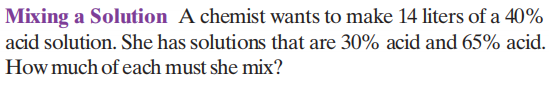### ¿Todavía tienes preguntas de matemáticas?

Pregunte a nuestros tutores expertos
Algebra
PreguntaA chemist wants to make $$14$$ liters of a $$40 \%$$ acid solution. She has solutions that are $$30 \%$$ acid and $$65 \%$$ acid. How much of each must she mix?